Next: Causality Up: FORMALISM Previous: Situations

## Fluents

A fluent is a function whose domain is the space Sit of situations. If the range of the function is (true, false), then it is called a propositional fluent. If its range is Sit, then it is called a situational fluent.

Fluents are often the values of functions. Thus raining(x) is a fluent such that raining(x)(s) is true if and only if it is raining at the place x in the situation s. We can also write this assertion as raining(x,s) making use of the well-known equivalence between a function of two variables and a function of the first variable whose value is a function of the second variable.

Suppose we wish to assert about a situation s that person p is in place x and that it is raining in place x. We may write this in several ways each of which has its uses:

1.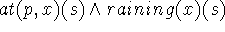. This corresponds to the definition given.

2.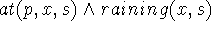. This is more conventional mathematically and a bit shorter.

3.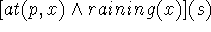. Here we are introducing a convention that operators applied to fluents give fluents whose values are computed by applying the logical operators to the values of the operand fluents, that is, if f and g are fluents then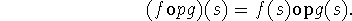4.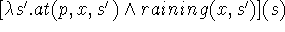. Here we have formed the composite fluent by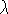-abstraction.

Here are some examples of fluents and expressions involving them:

1. time(s). This is the time associated with the situation s. It is essential to consider time as dependent on the situation as we shall sometimes wish to consider several different situations having the same time value, for example, the results of alternative courses of actions.

2. in(x,y,s). This asserts that x is in the location y in situation s. The fluent in may be taken as satisfying a kind of transitive law, namely: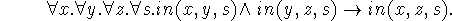We can also write this law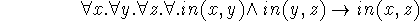where we have adopted the convention that a quantifier without a variable is applied to an implicit situation variable which is the (suppressed) argument of a propositional fluent that follows. Suppressing situation arguments in this way corresponds to the natural language convention of writing sentences like, `John was at home' or `John is at home' leaving understood the situations to which these assertions apply.

3. has(Monkey,Bananas,s). Here we introduce the convention that capitalized words denote proper names, for example, `Monkey' is the name of a particular individual. That the individual is a monkey is not asserted, so that the expression monkey(Monkey) may have to appear among the premisses of an argument. Needless to say, the reader has a right to feel that he has been given a hint that the individual Monkey will turn out to be a monkey. The above expression is to be taken as asserting that in the situation s the individual Monkey has the object Bananas. We shall, in the examples below, sometimes omit premisses such as monkey(Monkey), but in a complete system they would have to appear.Next: Causality Up: FORMALISM Previous: Situations

John McCarthy
Mon Apr 29 19:20:41 PDT 1996